Anúncio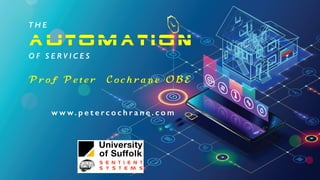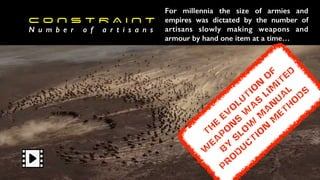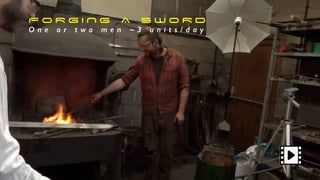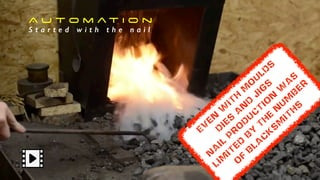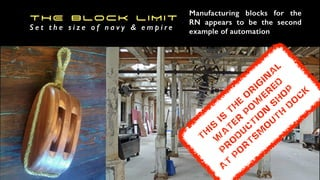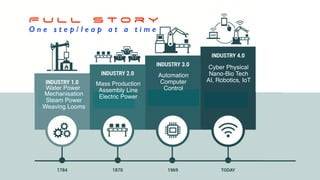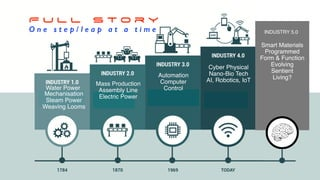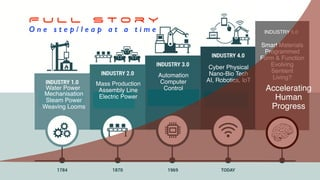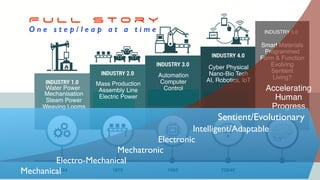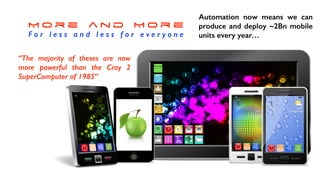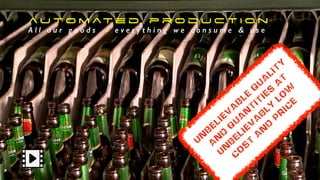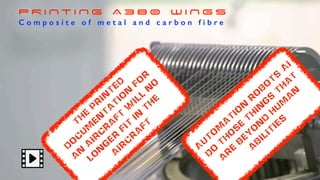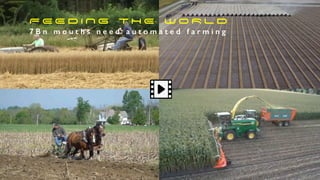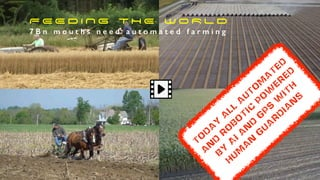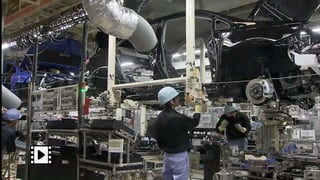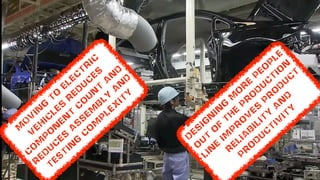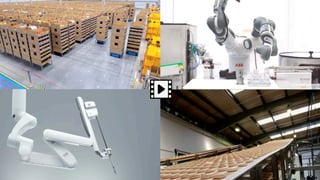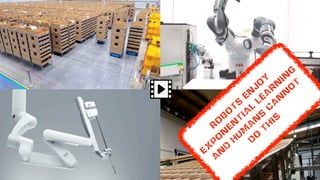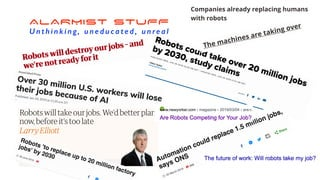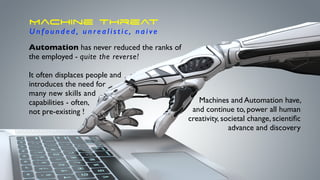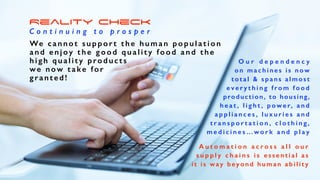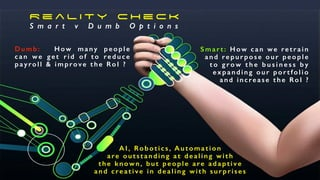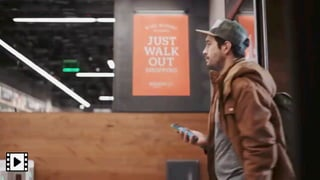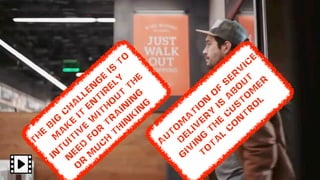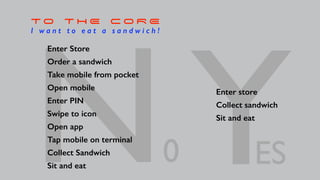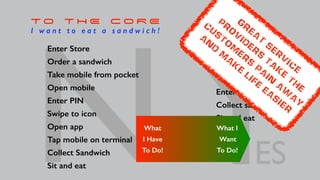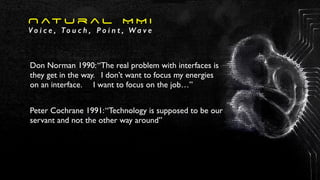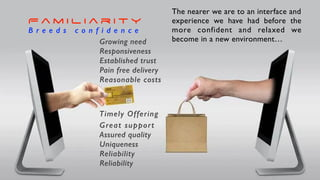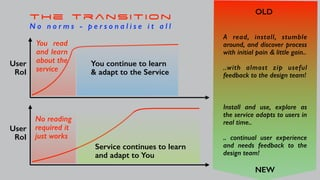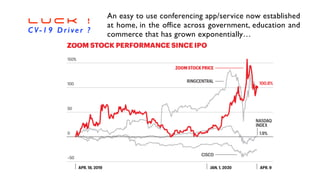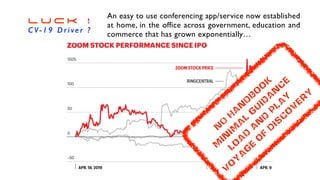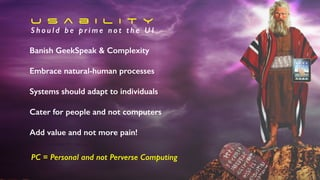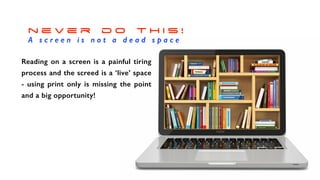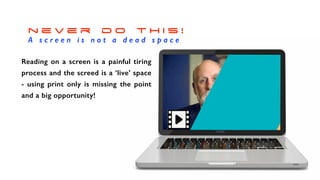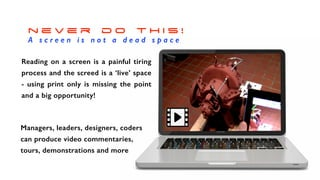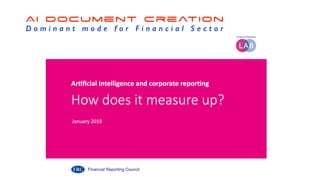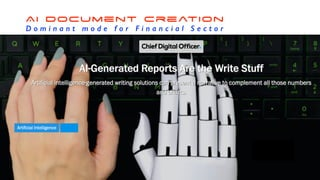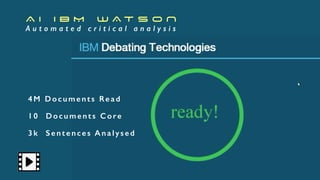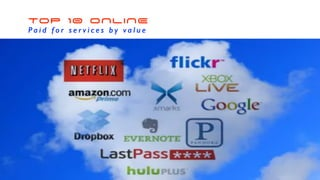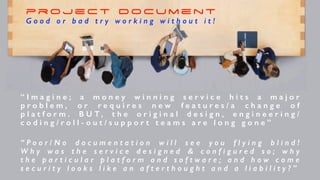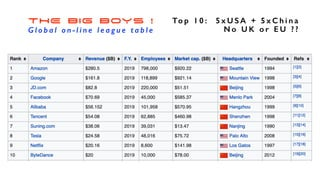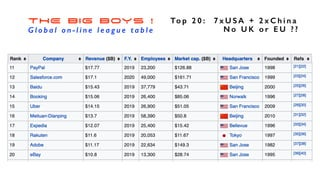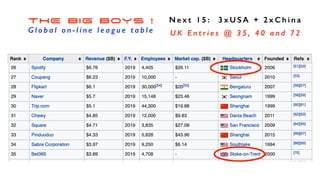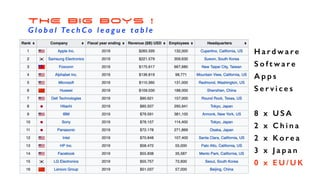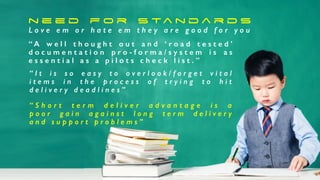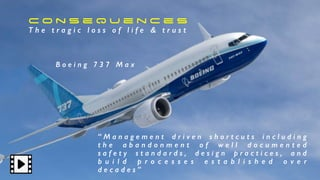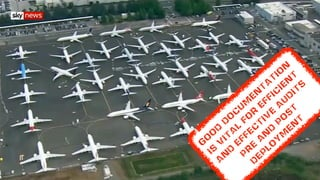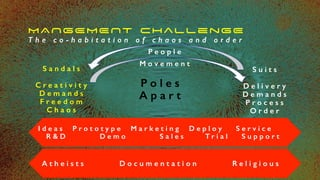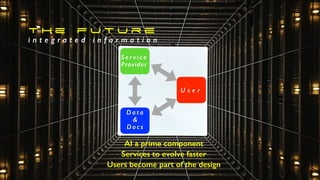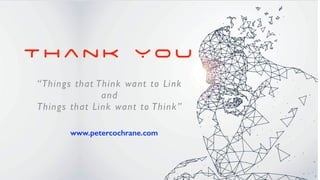1 de 54
Anúncio

### The Automation of Everything

1. T H E A U T O M A T I O N O F S E R V I C E S P r o f P e t e r C o c h r a n e O B E w w w. p e t e r c o c h r a n e . c o m
2. C O N S T R A I N T N u m b e r o f a r t i s a n s For millennia the size of armies and empires was dictated by the number of artisans slowly making weapons and armour by hand one item at a time… Th e ev o lu tio n o f w ea p o n s w a s lim ited b y s lo w m a n u a l p r o d u c tio n m eth o d s
3. F O R G I N G A S W O R D O n e o r t w o m e n ~ 3 u n i t s / d a y
4. A u t o m a t i o n S t a r t e d w i t h t h e n a i l Ev en w ith M o u ld s d ies a n d Jig s N a il p r o d u c tio n w a s lim ited b y th e n u m b er o f b la c k s m ith s
5. THE Block LIMIT S e t t h e s i z e o f n a v y & e m p i r e Manufacturing blocks for the RN appears to be the second example of automation Th is is th e o r ig in a l w a ter p o w er ed P r o d u c tio n s h o p A t P o r ts m o u th D o c k
6. Mechanisation Steam Power Weaving Looms Cyber Physical Nano-Bio Tech AI, Robotics, IoT Mass Production Assembly Line Electric Power Automation Computer ControlWater Power F U L L S t o r y O n e s t e p / l e a p a t a t i m e
7. Mechanisation Steam Power Weaving Looms Cyber Physical Nano-Bio Tech AI, Robotics, IoT Mass Production Assembly Line Electric Power Automation Computer ControlWater Power F U L L S t o r y O n e s t e p / l e a p a t a t i m e INDUSTRY 5.0 Smart Materials Programmed Form & Function Evolving Sentient Living?
8. Mechanisation Steam Power Weaving Looms Cyber Physical Nano-Bio Tech AI, Robotics, IoT Mass Production Assembly Line Electric Power Automation Computer ControlWater Power F U L L S t o r y O n e s t e p / l e a p a t a t i m e INDUSTRY 5.0 Smart Materials Programmed Form & Function Evolving Sentient Living? Accelerating Human Progress
9. Mechanisation Steam Power Weaving Looms Cyber Physical Nano-Bio Tech AI, Robotics, IoT Mass Production Assembly Line Electric Power Automation Computer ControlWater Power F U L L S t o r y O n e s t e p / l e a p a t a t i m e INDUSTRY 5.0 Smart Materials Programmed Form & Function Evolving Sentient Living? Accelerating Human Progress Sentient/Evolutionary Intelligent/Adaptable Electronic Mechatronic Electro-Mechanical Mechanical
10. M o r e a n d M o r e F o r l e s s a n d l e s s f o r e v e r y o n e Automation now means we can produce and deploy ~2Bn mobile units every year… “The majority of theses are now more powerful than the Cray 2 SuperComputer of 1985”
11. a u t o m at e d p r o d u c t i o n A l l o u r g o o d s - e v e r y t h i n g w e c o n s u m e & u s e U n b eliev a b le q u a lity a n d q u a n tities a t U n b eliev a b ly lo w c o s t a n d p r ic e
12. P R I N T I N G A 3 8 0 W I N G S C o m p o s i t e o f m e t a l a n d c a r b o n f i b r e Th e p r in ted d o c u m en ta tio n fo r a n a ir c r a ft w ill n o lo n g er fit in th e a ir c r a ft A u to m a tio n r o b o ts A I d o th o s e th in g s th a t a r e b ey o n d h u m a n a b ilities
13. F E E D I N G T H E W O R L D 7 B n m o u t h s n e e d a u t o m a t e d f a r m i n g
14. F E E D I N G T H E W O R L D 7 B n m o u t h s n e e d a u t o m a t e d f a r m i n g To d a y a ll a u to m a ted a n d r o b o tic p o w er ed b y A I a n d G P S w ith h u m a n g u a r d ia n s
15. H u m a n - R o b o t M i x P e o p l e d o w h a t a u t o m a t i o n c a n ’ t
16. H u m a n - R o b o t M i x P e o p l e d o w h a t a u t o m a t i o n c a n ’ t D es ig n in g m o r e p eo p le o u t o f th e p r o d u c tio n lin e im p r o v es p r o d u c t r elia b ility a n d p r o d u c tiv ity M O V IN g to elec tr ic v eh ic les r ed u c es c o m p o n en t c o u n t a n d r ed u c es a s s em b ly a n d Tes tin g c o m p lex ity
17. U B I Q U I T o u s W e w o u l d d i e w i t h o u t
18. U B I Q U I T o u s W e w o u l d d i e w i t h o u t r o b o ts en jo y ex p o n en tia l lea r n in g a n d h u m a n s c a n n o t d o th is
19. ALARMIST STUFF U n t h i n k i n g, u n e d u c a t e d , u n re a l
20. Machine Threat Unfounded, unrealistic, naive Automation has never reduced the ranks of the employed - quite the reverse! It often displaces people and introduces the need for many new skills and capabilities - often, not pre-existing ! Machines and Automation have, and continue to, power all human creativity, societal change, scientiﬁc advance and discovery
21. Reality Check C o n t i n u i n g t o p r o s p e r We cannot support the human population and enjoy the good quality food and the h i gh q ua l it y products we now take for g r a n t e d ! O u r d e p e n d e n c y o n m a c h i n e s i s n o w t o t a l & s p a n s a l m o s t e v e r y t h i n g f r o m f o o d production, to housing, h e a t , l i g h t , p o w e r, a n d a p p l i a n c e s , l u x u r i e s a n d t r a n s p o r t a t i o n , c l o t h i n g , m e d i c i n e s …w o r k a n d p l a y A u t o m a t i o n a c r o s s a l l o u r s u p p l y c h a i n s i s e s s e n t i a l a s it is wa y beyond hu ma n a bilit y
22. R e a l i t y C h e c k S m a r t v D u m b O p t i o n s Dumb: H o w m a n y p e o p l e can we get rid of to reduce payroll & improve the RoI ? S m a r t : How can we retrain and repurpose our people t o g r o w t h e b u s i n e s s b y e x p a n d i n g o u r p o r t f o l i o and increase the RoI ? A I , Ro b o t i c s , A u t o m a t i o n are outstanding at dealing with t h e k n o w n , b u t p e o p l e a re a d a p t i v e and creative in dealing with surprises
23. N O C O N S T R A I N T S L i m i t e d o n l y b y o u r i m a g i n a t i o n
24. N O C O N S T R A I N T S L i m i t e d o n l y b y o u r i m a g i n a t i o n A u to m a tio n o f s er v ic e d eliv er y is a b o u t g iv in g th e c u s to m er to ta l c o n tr o l tH e B ig c h a llen g e is to m a k e it en tir ely in tu itiv e w ith o u t th e n eed fo r tr a in in g o r m u c h th in k in g
25. YESN0 Enter Store Order a sandwich Take mobile from pocket Open mobile Enter PIN Swipe to icon Open app Tap mobile on terminal Collect Sandwich Sit and eat Enter store Collect sandwich Sit and eat T O T H E C O R E I w a n t t o e a t a s a n d w i c h !
26. YESN0 Enter Store Order a sandwich Take mobile from pocket Open mobile Enter PIN Swipe to icon Open app Tap mobile on terminal Collect Sandwich Sit and eat Enter store Collect sandwich Sit and eat T O T H E C O R E I w a n t t o e a t a s a n d w i c h ! What I Have To Do! What I Want To Do! g r ea t ser v ic e pr o v id er s ta k e th e c usto m er s pa in a w a y a n d m a k e life ea sier
27. N at u r a l M M I V o i c e , To u c h , P o i n t , W a v e Don Norman 1990:“The real problem with interfaces is they get in the way. I don’t want to focus my energies on an interface. I want to focus on the job…” Peter Cochrane 1991:“Technology is supposed to be our servant and not the other way around”
28. F a m i l i a r i t y B r e e d s c o n f i d e n c e The nearer we are to an interface and experience we have had before the more confident and relaxed we become in a new environment…Growing need Responsiveness Established trust Pain free delivery Reasonable costs Timely Offering Great support Assured quality Uniqueness Reliability Reliability
29. OLD NEW the transition N o n o r m s - p e r s o n a l i s e i t a l l User RoI User RoI You continue to learn & adapt to the Service Service continues to learn and adapt to You You read and learn about the service No reading required it just works A read, install, stumble around, and discover process with initial pain & little gain.. ..with almost zip useful feedback to the design team! Install and use, explore as the service adapts to users in real time.. .. continual user experience and needs feedback to the design team!
30. Z L U C K ! CV-19 Driver ? An easy to use conferencing app/service now established at home, in the office across government, education and commerce that has grown exponentially…
31. Z L U C K ! CV-19 Driver ? An easy to use conferencing app/service now established at home, in the office across government, education and commerce that has grown exponentially… N O H a n d b o o k M in im a l g u id a n c e lo a d a n d p la y v o y a g e o f d is c o v er y
32. h a n d b o o k s U s e r G u i d e s / T u t o r i a l s An admission of poor design - apps/services that should be as intuitive as possible - with ‘boot’ to a basic mode and ready for the customer to play/use/apply! The extent of a design failure is inversely proportional to the weight of a handbook and/or the length of a training tutorial + the number of calls for help! Customer experiences like this lose business & reputation!
33. u s a b i l i t y S h o u l d b e p r i m e n o t t h e U I PC = Personal and not Perverse Computing Banish GeekSpeak & Complexity Embrace natural-human processes Systems should adapt to individuals Cater for people and not computers Add value and not more pain!
34. r e a d i n g ? A m o s t i n e f f i c i e n t m o d e Taking paper documents and putting them on a screen is a really bad idea! Manual creation of written documents is an even worse idea! If a picture is worth 1k words An animated picture is worth 1M 3D AR interaction is worth 1G
35. n e v e r d o t h i s ! A s c r e e n i s n o t a d e a d s p a c e Reading on a screen is a painful tiring process and the screed is a ‘live’ space - using print only is missing the point and a big opportunity!
36. n e v e r d o t h i s ! A s c r e e n i s n o t a d e a d s p a c e Reading on a screen is a painful tiring process and the screed is a ‘live’ space - using print only is missing the point and a big opportunity!
37. n e v e r d o t h i s ! A s c r e e n i s n o t a d e a d s p a c e Reading on a screen is a painful tiring process and the screed is a ‘live’ space - using print only is missing the point and a big opportunity! Managers, leaders, designers, coders can produce video commentaries, tours, demonstrations and more
38. AI Document Creation D o m i n a n t m o d e f o r F i n a n c i a l S e c t o r
39. AI Document Creation D o m i n a n t m o d e f o r F i n a n c i a l S e c t o r
40. AI Document Creation D o m i n a n t m o d e f o r F i n a n c i a l S e c t o r M o s t fin a n c ia l r ep o r ts a r e n o w w r itten /c o m p iled a n d p u b lis h ed b y A I M o s t d efin itely c o m in g to y o u r s ec to r v er y s o o n a u to m a ted d o c u m en ta tio n c r ea tio n m a y n o t b e a d r ea m fo r s o v er y lo n g
41. A I I B M W A T S O N A u t o m a t e d c r i t i c a l a n a l y s i s 4M Documents Read 10 Documents Core 3k Sentences Analysed
42. TOP 10 ONLINE P a i d f o r s e r v i c e s b y v a l u e
43. projec t Docum ent G o o d o r b a d t r y w o r k i n g w i t h o u t i t ! “ I m a g i n e ; a m o n e y w i n n i n g s e r v i c e h i t s a m a j o r p r o b l e m , o r r e q u i r e s n e w f e a t u r e s / a c h a n g e o f p l a t f o r m . B U T , t h e o r i g i n a l d e s i g n , e n g i n e e r i n g / c o d i n g / r o l l - o u t / s u p p o r t t e a m s a r e l o n g g o n e ” “ P o o r / N o d o c u m e n t a t i o n w i l l s e e y o u f l y i n g b l i n d ! W h y w a s t h e s e r v i c e d e s i g n e d & c o n f i g u r e d s o ; w h y t h e p a r t i c u l a r p l a t f o r m a n d s o f t w a r e ; a n d h o w c o m e s e c u r i t y l o o k s l i k e a n a f t e r t h o u g h t a n d a l i a b i l i t y ? ”
44. THE BIG BOYS ! G l o b a l o n - l i n e l e a g u e t a b l e To p 1 0 : 5 x U S A + 5 x C h i n a N o U K o r E U ? ?
45. THE BIG BOYS ! G l o b a l o n - l i n e l e a g u e t a b l e To p 2 0 : 7 x U S A + 2 x C h i n a N o U K o r E U ? ?
46. THE BIG BOYS ! G l o b a l o n - l i n e l e a g u e t a b l e N e x t 1 5 : 3 x U S A + 2 x C h i n a U K E n t r i e s @ 3 5 , 4 0 a n d 7 2
47. THE BIG BOYS ! G l o b a l Te c h C o l e a g u e t a b l e H a r d w a r e S o f t w a r e A p p s S e r v i c e s 8 x U S A 2 x C h i n a 2 x K o r e a 3 x J a p a n 0 x E U / U K
48. n e e d f o r s ta n d a r d s L o v e e m o r h a t e e m t h e y a r e g o o d f o r y o u “ A w e l l t h o u g h t o u t a n d ‘ r o a d t e s t e d ’ d o c u m e n t a t i o n p r o - f o r m a / s y s t e m i s a s e s s e n t i a l a s a p i l o t s c h e c k l i s t . ” “ I t i s s o e a s y t o o v e r l o o k / f o r g e t v i t a l i t e m s i n t h e p r o c e s s o f t r y i n g t o h i t d e l i v e r y d e a d l i n e s ” “ S h o r t t e r m d e l i v e r a d v a n t a g e i s a p o o r g a i n a g a i n s t l o n g t e r m d e l i v e r y a n d s u p p o r t p r o b l e m s ”
49. C o n s e q u e n c e s T h e t r a g i c l o s s o f l i f e & t r u s t “ M a n a g e m e n t d r i v e n s h o r t c u t s i n c l u d i n g t h e a b a n d o n m e n t o f w e l l d o c u m e n t e d s a f e t y s t a n d a r d s , d e s i g n p r a c t i c e s , a n d b u i l d p r o c e s s e s e s t a b l i s h e d o v e r d e c a d e s ” B o e i n g 7 3 7 M a x
50. C o n s e q u e n c e s T h e t r a g i c l o s s o f l i f e & t r u s t “ M a n a g e m e n t d r i v e n s h o r t c u t s i n c l u d i n g t h e a b a n d o n m e n t o f w e l l d o c u m e n t e d s a f e t y s t a n d a r d s , d e s i g n p r a c t i c e s , a n d b u i l d p r o c e s s e s e s t a b l i s h e d o v e r d e c a d e s ” B o e i n g 7 3 7 M a x g o o d d o c u m en ta tio n is v ita l fo r effic ien t a n d effec tiv e a u d its p r e a n d p o s t d ep lo y m en t
51. MANGEMENT challenGE T h e c o - h a b i t a t i o n o f c h a o s a n d o r d e r S a n d a l s C r e a t i v i t y D e m a n d s F r e e d o m C h a o s S u i t s D e l i v e r y D e m a n d s P r o c e s s O r d e r P o l e s A p a r t I d e a s P r o t o t y p e M a r k e t i n g D e p l o y S e r v i c e R & D D e m o S a l e s T r i a l S u p p o r t P e o p l e M o v e m e n t A t h e i s t s D o c u m e n t a t i o n R e l i g i o u s
52. t h e f u t u r e i n t e g r a t e d i n f o r m a t i o n U s e r S e r v i c e Provider D a t a & D o c s AI a prime component Services to evolve faster Users become part of the design
53. T h a n k Y o u www.petercochrane.com “Things that Think want to Link and Things that Link want to Think”
Anúncio